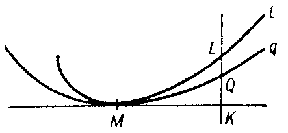# Osculation

of a curve \$q\$ with a curve \$l\$ at a given point \$M\$

A geometrical concept, meaning that \$q\$ has contact of maximal order with \$l\$ at \$M\$ in comparison with any curve in some given family of curves \$\{q\}\$ including \$q\$. The order of contact of \$q\$ and \$l\$ is said to be equal to \$n\$ if the segment \$QL\$ is a variable of \$(n+1)\$-st order of smallness with respect to \$MK\$ (see Fig., where \$QL\$ is perpendicular to the common tangent of \$q\$ and \$l\$ at \$M\$).Figure: o070590a

Thus, of all the curves in \$\{q\}\$, the curve having osculation with \$l\$ is the one which is most closely adjacent to \$l\$ (that is, for which \$QL\$ has maximal order of smallness). The curve in \$\{q\}\$ having osculation with \$l\$ at a given point \$M\$ is called the osculating curve of the given family at this point. E.g., the osculating circle of \$l\$ at \$M\$ is the circle having maximal order of contact with \$l\$ at \$M\$ in comparison with any other circle.

Similarly one can define the concept of osculation of a surface \$S\$ in a given family of surfaces \$\{S\}\$ with a curve \$l\$ (or with a surface) at some point \$M\$ of it. Here the order of contact is defined similarly, except that one must examine the tangent plane of \$S\$ at \$M\$ instead of the tangent line \$MK\$ in the figure.

How to Cite This Entry:
Osculation. Encyclopedia of Mathematics. URL: http://encyclopediaofmath.org/index.php?title=Osculation&oldid=31966
This article was adapted from an original article by BSE-3 (originator), which appeared in Encyclopedia of Mathematics - ISBN 1402006098. See original article